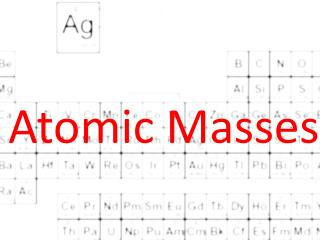DownloadDownload PresentationAtomic Masses

# Atomic Masses

Download Presentation## Atomic Masses

- - - - - - - - - - - - - - - - - - - - - - - - - - - E N D - - - - - - - - - - - - - - - - - - - - - - - - - - -
##### Presentation Transcript

1. Atomic Masses

2. Mass of Atoms • The mass of atoms are very small • Oxygen-16 has a mass of 2.657 x 10-23 • It is more convenient to use a relative atomic mass • The standard for this new relative mass is carbon-12 • Carbon-12 has exactly 12 atomic mass units(amu) • Or ½ of the mass of carbon-12 equaks one amu

3. Mass of Atoms • The mass of an electron is .0005486 amu • The mass of a proton is 1.007276 amu • The mass of a neutron is 1.008665 amu • The mass of oxygen-16 is 15.994915 amu • The mass of magnesium-24 is 23.985042 amu • The unit for atomic mass on the periodic table is in amu’s.

4. Average Atomic Mass • Average atomic mass: the weighted average of the atomic masses of naturally occurring isotopes of an element • Weighted average? • In a class your homework is 30% of the final grade, quizzes are 30% and tests are 40%. What is your final percentage if you have 89% on homework, 85% on quizzes and 79 % on tests?

5. Average Atomic Mass • Copper-63 has a mass of 62.929598 amu and is 69.17% abundant. Copper-65 has a mass of 64.927793 amu and is 30.83% abundant. What is the average atomic mass of copper? • Oxygen-16 is 99.762% abundant. Oxygen-17 is .038% abundant. And oxygen-18 is .200% abundant. What is the average atomic mass for oxygen?

6. The MOLE

7. The Mole • Mole: the amount of a substance that contains as many particles as there are in exactly 12g of carbon-12 • A mole is similar to a dozen

8. Avogadro’s Number • Avogadro’s number: the number of particles in exactly one mole of a pure substance • Avogadro’s number = 6.022x1023

9. The Mole • 1 mol = 6.022 x 1023 atoms • Look it’s a conversion factor! • Convert 2.56 mols of Ca to atoms • Convert 5.63 x 1022 atoms of Br to mols

10. Molar Mass • Molar Mass: The mass of one mole of a substance • Unit for molar mass is g/mol • Molar mass is numerically equal to the average atomic mass in amu

11. Molar Mass • Molar mass of lithium is 6.94 g/mol or 6.94 g 1 mol • Molar mass is a conversion factor • Convert 2.34 mol of Li to g • Convert 150 g of Pb to mol